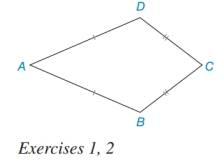Chapter 7.3, Problem 1E### Elementary Geometry for College St...

6th Edition
Daniel C. Alexander + 1 other
ISBN: 9781285195698

#### Solutions

Chapter
Section### Elementary Geometry for College St...

6th Edition
Daniel C. Alexander + 1 other
ISBN: 9781285195698
Textbook Problem
1 views

# Describe, if possible, how you would inscribe a circle within kite ABCD.To determine

To find:

The procedure to draw a circle inscribe a kite.

Explanation

Steps of construction to draw a circle inscribe a kite.

1) Construct the angle bisectors of BandC which intersect each other at O.

2) Draw a perpendicular from the centre O to side BC i.e., OM.

### Still sussing out bartleby?

Check out a sample textbook solution.

See a sample solution

#### The Solution to Your Study Problems

Bartleby provides explanations to thousands of textbook problems written by our experts, many with advanced degrees!

Get Started

#### Write the sum in expanded form. 4. i=46i3

Single Variable Calculus: Early Transcendentals, Volume I

#### Evaluate Evaluate the expression. 16. 250

Precalculus: Mathematics for Calculus (Standalone Book)

#### Identify and describe the steps of the scientific method.

Research Methods for the Behavioral Sciences (MindTap Course List)